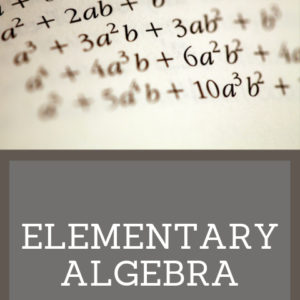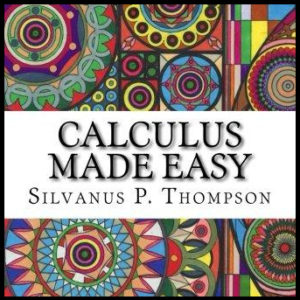Showing all 8 results

•\$10.00

Elementary Algebra is designed to meet the scope and sequence requirements of a a full year of elementary algebra. Each topic builds upon previously developed material to demonstrate the cohesiveness and structure of mathematics. Topics studied:

Arithmetic Review
Real Numbers
Algebraic Expressions & Equations
Linear Equations & Inequalities
Polynomials
Graphing Linear Equations & Inequalities
Rational Expressions
Roots, Radicals and Square Root Equations
Systems of Linear Equations

•\$3.00

Calculus Made Easy is a book on infinitesimal calculus originally published in 1910 by Silvanus P. Thompson, considered a classic and elegant introduction to the subject.

•\$3.50

Written in 1895, this text includes over 1000 exercises for practice. Topics covered include operations, factoring, fractions, complex fractions, solving equations, and solving simultaneous equations. Answers are provided at the end of the book.

•\$9.99

This algebra textbook teaches students the following:

• Pre-Algebra
• Solving Linear Equations
• Graphing
• Inequalities
• Systems of Equations
• Polynomials
• Factoring
• Rational Expressions
• Functions

•\$19.99

Complete High School Geometry course

•\$14.99

••